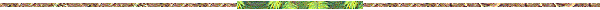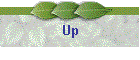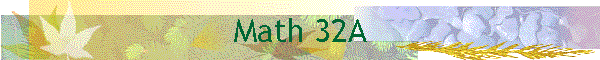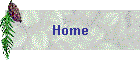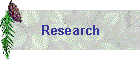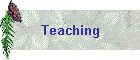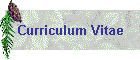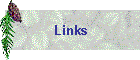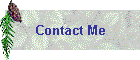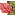Course: Math 32A, Calculus of Several VariablesText: Jerrold Marsden & Anthony Tromba, Vector Calculus, 5th Ed., FreemanSchedule of Lectures: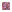Section 16.1-2: Integration in two variables, iterated integralsSection 16.3: Integration over non-rectangular regionsSection 16.4: Integration in polar coordinatesSection 16.5: Applications of double integralsSection 16.6: Surface areaSection 16.7: Triple integralsSection 16.8: Integration in cylindrical and spherical coordinatesSection 16.9: Change of variablesSection 17.1: Vector fieldsSection 17.2: Line integralsSection 17.3: Fundamental theorem for line integralsSection 17.4: Green's theoremSection 17.5: Divergence and curlSection 17.6: Parametric surfaces and their areasSection 17.7: Surface integralsSection 17.8: Stokes' theoremSection 17.9: Gauss's theorem